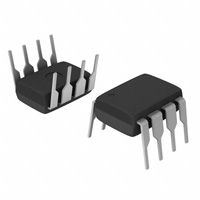< id="6e2kg">< id="6e2kg">< id="6e2kg">< id="6e2kg">< id="6e2kg">< id="6e2kg">< id="6e2kg">< id="6e2kg">< id="6e2kg">< id="6e2kg">< id="6e2kg">< id="6e2kg">< id="6e2kg">< id="6e2kg">< id="6e2kg">< id="6e2kg">< id="6e2kg">< id="6e2kg">< id="6e2kg">< id="6e2kg">< id="6e2kg">< id="6e2kg">< id="6e2kg">< id="6e2kg">< id="6e2kg">< id="6e2kg">< id="6e2kg">< id="6e2kg">< id="6e2kg">< id="6e2kg">< id="6e2kg">< id="6e2kg">< id="6e2kg">< id="6e2kg">< id="6e2kg">< id="6e2kg">< id="6e2kg">< id="6e2kg">< id="6e2kg">< id="6e2kg">< id="6e2kg">< id="6e2kg">< id="6e2kg">< id="6e2kg">< id="6e2kg">< id="6e2kg">< id="6e2kg">< id="6e2kg">< id="6e2kg">< id="6e2kg"> < id="6e2kg">< id="6e2kg">< id="6e2kg">< id="6e2kg">< id="6e2kg">< id="6e2kg">< id="6e2kg">< id="6e2kg">< id="6e2kg">< id="6e2kg">< id="6e2kg">< id="6e2kg">< id="6e2kg">< id="6e2kg">< id="6e2kg">< id="6e2kg">< id="6e2kg">< id="6e2kg">< id="6e2kg">< id="6e2kg">< id="6e2kg">< id="6e2kg">< id="6e2kg">< id="6e2kg">< id="6e2kg">< id="6e2kg">< id="6e2kg">< id="6e2kg">< id="6e2kg">< id="6e2kg">< id="6e2kg">< id="6e2kg">< id="6e2kg">< id="6e2kg">< id="6e2kg">< id="6e2kg">< id="6e2kg">< id="6e2kg">< id="6e2kg">< id="6e2kg">< id="6e2kg">< id="6e2kg">< id="6e2kg">< id="6e2kg">< id="6e2kg">< id="6e2kg">< id="6e2kg">< id="6e2kg">< id="6e2kg">< id="6e2kg"> < id="6e2kg">< id="6e2kg">< id="6e2kg">< id="6e2kg">< id="6e2kg">< id="6e2kg">< id="6e2kg">< id="6e2kg">< id="6e2kg">< id="6e2kg">< id="6e2kg">< id="6e2kg">< id="6e2kg">< id="6e2kg">< id="6e2kg">< id="6e2kg">< id="6e2kg">< id="6e2kg">< id="6e2kg">< id="6e2kg">< id="6e2kg">< id="6e2kg">< id="6e2kg">< id="6e2kg">< id="6e2kg">< id="6e2kg">< id="6e2kg">< id="6e2kg">< id="6e2kg">< id="6e2kg">< id="6e2kg">< id="6e2kg">< id="6e2kg">< id="6e2kg">< id="6e2kg">< id="6e2kg">< id="6e2kg">< id="6e2kg">< id="6e2kg">< id="6e2kg">< id="6e2kg">< id="6e2kg">< id="6e2kg">< id="6e2kg">< id="6e2kg">< id="6e2kg">< id="6e2kg">< id="6e2kg">< id="6e2kg">< id="6e2kg">型号： AT24C02-10PI-2.7 厂商：Atmel 分类： 集成电路 ， 存储器 描述： IC eeprom 2kbit 400khz 8dip PDF： 预览 报错 收藏 赞

Datasheet下载地址

 本地下载 >> 第三方平台下载 >>

AT24C02-10PI-2.7的详细信息

Datasheets： 50 Integrated Circuits (ICs) Memory - Tube EEPROMs - Serial EEPROM 2K (256 x 8) 400kHz I2C, 2-Wire Serial 2.7 V ~ 5.5 V -40°C ~ 85°C 8-DIP (0.300", 7.62mm) 8-PDIP
Features
? Low-voltage and Standard-voltage Operation
– 5.0 (VCC = 4.5V to 5.5V)
– 2.7 (VCC = 2.7V to 5.5V)
– 2.5 (VCC = 2.5V to 5.5V)
– 1.8 (VCC = 1.8V to 5.5V)
? Internally Organized 128 x 8 (1K), 256 x 8 (2K), 512 x 8 (4K),
1024 x 8 (8K) or 2048 x 8 (16K)
? 2-wire Serial Interface
? Schmitt Trigger, Filtered Inputs for Noise Suppression
? Bi-directional Data Transfer Protocol
? 100 kHz (1.8V, 2.5V, 2.7V) and 400 kHz (5V) Compatibility
? Write Protect Pin for Hardware Data Protection
? 8-byte Page (1K, 2K), 16-byte Page (4K, 8K, 16K) Write Modes
? Partial Page Writes are Allowed
2-wire
Serial EEPROM
1K (128 x 8)
? Self-timed Write Cycle (10 ms max)
? High-reliability
– Endurance: 1 Million Write Cycles
– Data Retention: 100 Years
2K (256 x 8)
? Automotive Grade and Extended Temperature Devices Available
? 8-lead JEDEC SOIC, 8-pin PDIP and 8-lead TSSOP Packages
4K (512 x 8)
8K (1024 x 8)
16K (2048 x 8)
Description
The AT24C01A/02/04/08/16 provides 1024/2048/4096/8192/16384 bits of serial elec-
trically erasable and programmable read-only memory (EEPROM) organized as
128/256/512/1024/2048 words of 8 bits each. The device is optimized for use in many
industrial and commercial applications where low-power and low-voltage operation
are essential. The AT24C01A/02/04/08/16 is available in space-saving 8-pin PDIP,
JEDEC SOIC (AT24C01A/02/04/08/16) packages and is accessed via a 2-wire serial
interface. In addition, the entire family is available in 5.0V (4.5V to 5.5V), 2.7V (2.7V to
5.5V), 2.5V (2.5V to 5.5V) and 1.8V (1.8V to 5.5V) versions.
AT24C01A
AT24C02
AT24C04
AT24C08
AT24C16
Pin Configurations
Pin Name Function
A0
A1
1
2
3
4
8
7
6
5
VCC
WP
A0 - A2
SDA
SCL
WP
A2
SCL
SDA
GND
Serial Data
Serial Clock Input
Write Protect
No Connect
NC
8-pin PDIP
A0
1
2
3
4
8
7
6
5
VCC
WP
A0
1
2
3
4
8
7
6
5
VCC
A1
A2
A1
A2
WP
SCL
SDA
SCL
SDA
GND
GND
Rev. 0180F–06/01